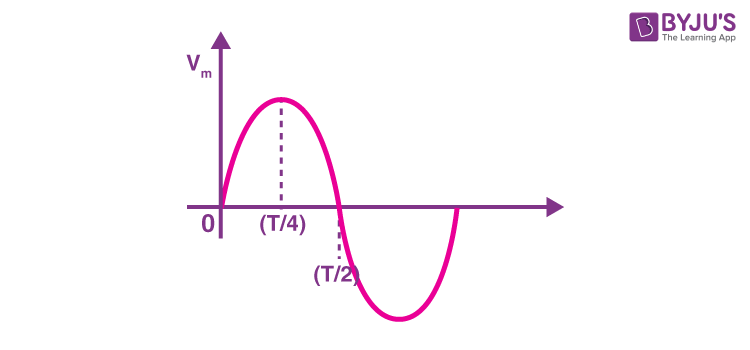# An Ac Source Is Rated At 220V, 50Hz. The Time Taken For Voltage To Change From Its Peak Value To Zero Is____.

The time taken for voltage to change from its peak value to zero is:$$(\frac{T}{2}) – (\frac{T}{4}) = (\frac{2T}{8}) = (\frac{T}{4})$$

As given:

f= 50 Hz

$$\Rightarrow T = \frac{1}{50}$$ sec

$$\Rightarrow T = \frac{T}{4}$$ $$\Rightarrow\frac{1}{50 * 4}$$ $$\Rightarrow \frac{1}{200}$$ $$\Rightarrow 5 * 10^{-3}$$ sec

Therefore, the time taken for voltage to change from its peak value to zero is: $$5 * 10^{-3}$$sec

Explore more such questions and answers at BYJU’S.(0)(0)